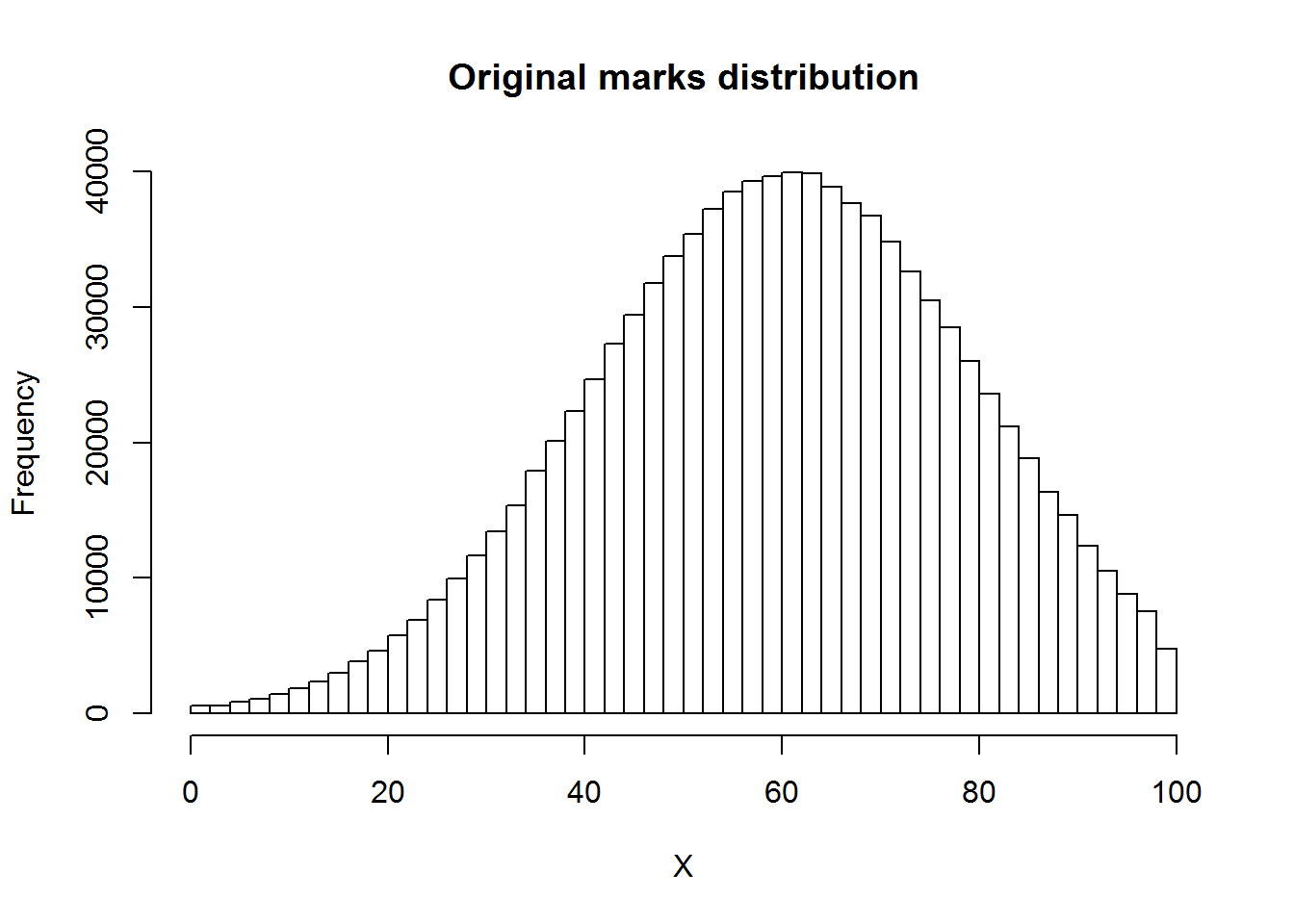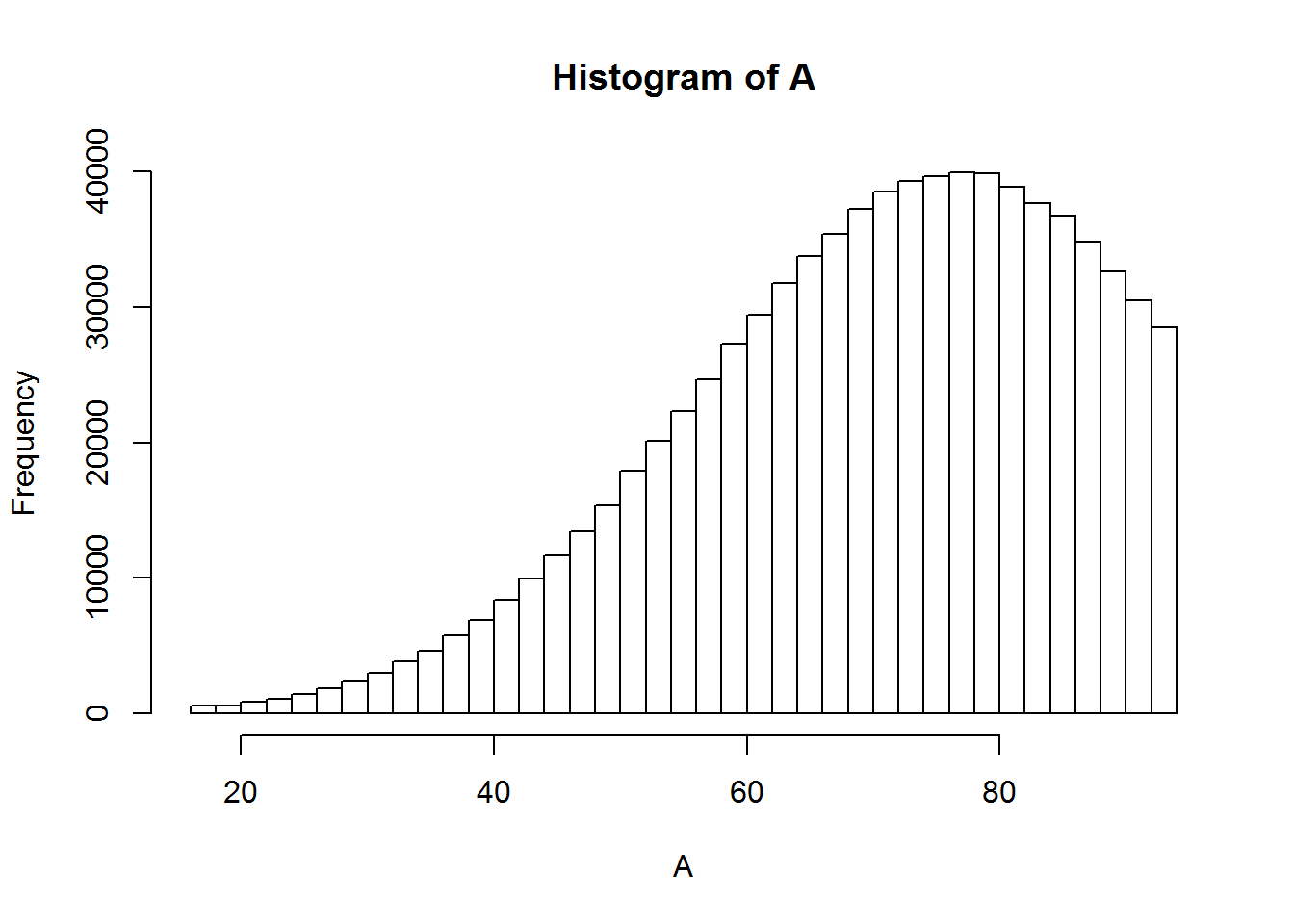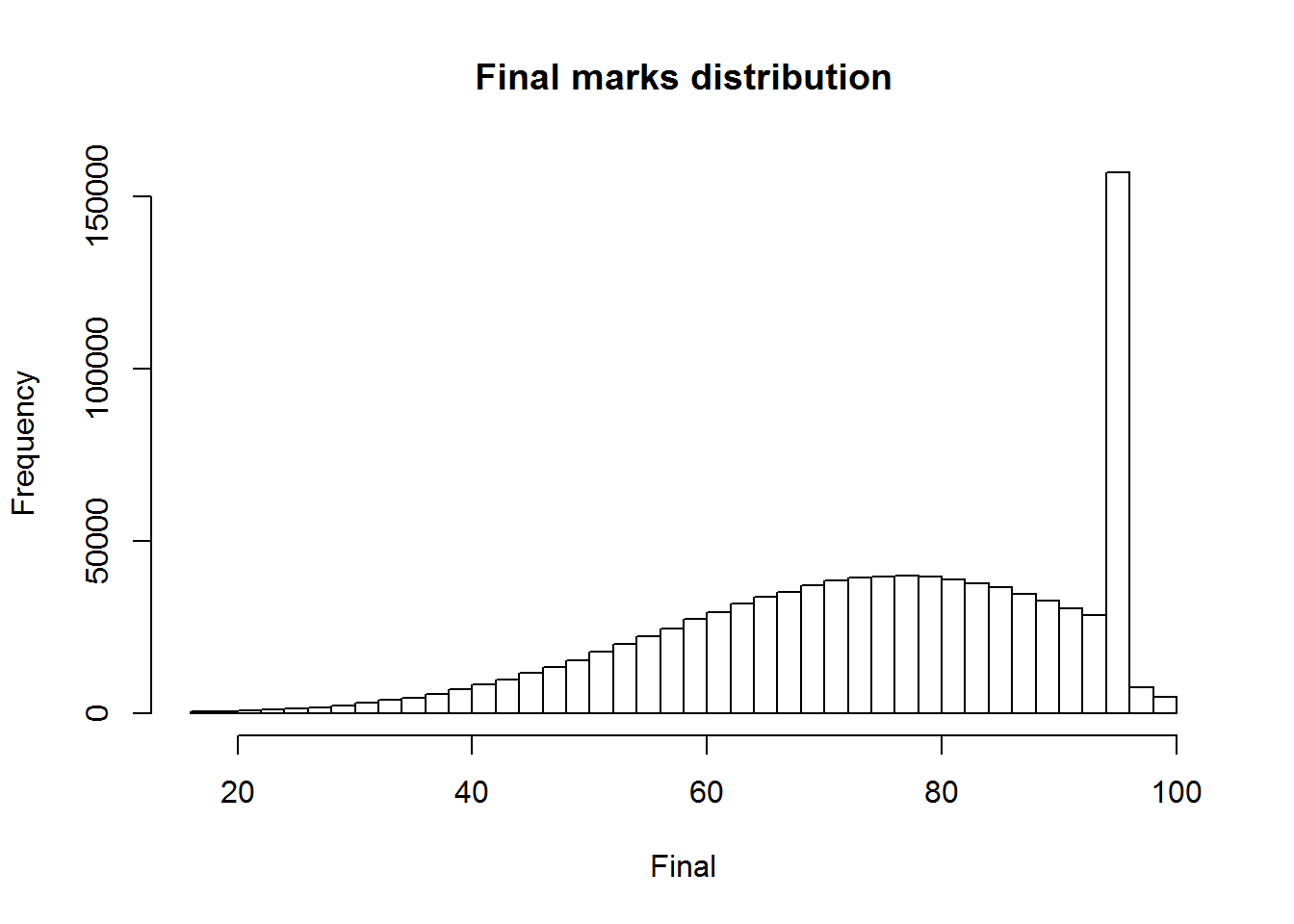Let us simulate a dataset of 1 million students with given mean and sd.

X = rnorm(1000000,61,20)

Now let us Truncate the distribution between 0 and 100 and round to nearest integer and look at summary

X = X[X<=100 & X>= 0]

X= round(X,digits=0)

summary(X)
##    Min. 1st Qu.  Median    Mean 3rd Qu.    Max.
##    0.00   47.00   60.00   59.82   73.00  100.00

Let’s look at histogram of original marks

hist(X ,breaks=50,main = "Original marks distribution")Now let’s look at percent of people with less than 33 marks

mean(X<33)
##  0.07850118

Now let’s implement our moderation strategy where 16 added to every mark less than 78 and those greater than 95 left untouched

Y = X[X>=95] # subset greater than 95 not moderated

A = X[X<=78] # subset less than 78 all added 16 numbers
A=A+16

hist(A,breaks=50)B=X[X>=79 & X <=95] # subset between 78 and 94 all made 95

B = rep(95,length(B))
Final = c(Y,A,B) # adding all subset again for final curve.

Generating histogram again of final marks post moderation

hist(Final,breaks = 50, main="Final marks distribution")summary of inflated mark distribution

summary(Final)
##    Min. 1st Qu.  Median    Mean 3rd Qu.    Max.
##   16.00   63.00   76.00   74.66   89.00  100.00